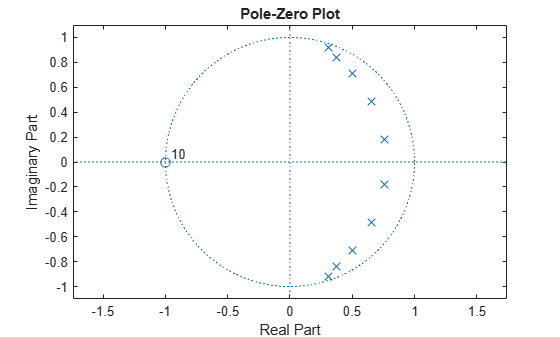# isminphase

Verify that discrete-time filter System object is minimum phase

## Syntax

``flag = isminphase(sysobj)``
``flag = isminphase(sysobj,tol)``
``flag = isminphase(___,'Arithmetic',arithType)``

## Description

example

````flag = isminphase(sysobj)` returns `true` if the filter System object™ has minimum phase.```
````flag = isminphase(sysobj,tol)` uses the tolerance `tol` to determine when two numbers are close enough to be considered equal. If not specified, `tol` defaults to `eps^(2/3)`.```
````flag = isminphase(___,'Arithmetic',arithType)` analyzes the filter System object based on the arithmetic specified in the `arithType` input using either of the previous syntaxes.For more input options, see `isminphase` in Signal Processing Toolbox™.```

## Examples

collapse all

Design a Chebyshev Type I IIR filter and determine if the filter has minimum phase and is stable.

Using the `fdesign.lowpass` and `design` functions, design a Chebyshev Type I IIR filter with a passband ripple of 0.5 dB and a 3 dB cutoff frequency at 9600 Hz.

```Fs = 48000; % Sampling frequency of input signal d = fdesign.lowpass('N,F3dB,Ap', 10, 9600, .5, Fs); filt = design(d,'cheby1','Systemobject',true)```
```filt = dsp.SOSFilter with properties: Structure: 'Direct form II' CoefficientSource: 'Property' Numerator: [5x3 double] Denominator: [5x3 double] HasScaleValues: true ScaleValues: [0.3318 0.2750 0.1876 0.0904 0.0225 0.9441] Show all properties ```

Using the `isminphase` function, determine if the filter has minimum phase.

`isminphase(filt)`
```ans = logical 1 ```

Verify the location of poles and zeros of the filter transfer function on the z-plane. By definition, the poles and zeros of the minimum phase filter must be on or inside the unit circle.

`zplane(filt)`All minimum phase filters are stable. To verify if the designed filter is stable, use the `isstable` function.

`isstable(filt)`
```ans = logical 1 ```

## Input Arguments

collapse all

Tolerance value to determine when two numbers are close enough to be considered equal, specified as a positive scalar. If not specified, `tol` defaults to `eps^(2/3)`.

Arithmetic used in the filter analysis, specified as `'double'`, `'single'`, or `'Fixed'`. When the arithmetic input is not specified and the filter System object is unlocked, the analysis tool assumes a double-precision filter. When the arithmetic input is not specified and the System object is locked, the function performs the analysis based on the data type of the locked input.

The `'Fixed'` value applies to filter System objects with fixed-point properties only.

When the `'Arithmetic'` input argument is specified as `'Fixed'` and the filter object has the data type of the coefficients set to `'Same word length as input'`, the arithmetic analysis depends on whether the System object is unlocked or locked.

• unlocked –– The analysis object function cannot determine the coefficients data type. The function assumes that the coefficients data type is signed, has a 16-bit word length, and is auto scaled. The function performs fixed-point analysis based on this assumption.

• locked –– When the input data type is `'double'` or `'single'`, the analysis object function cannot determine the coefficients data type. The function assumes that the data type of the coefficients is signed, has a 16-bit word length, and is auto scaled. The function performs fixed-point analysis based on this assumption.

To check if the System object is locked or unlocked, use the `isLocked` function.

When the arithmetic input is specified as `'Fixed'` and the filter object has the data type of the coefficients set to a custom numeric type, the object function performs fixed-point analysis based on the custom numeric data type.

## Output Arguments

collapse all

Flag to determine if the filter has minimum phase, returned as a logical:

• `1` –– Filter has minimum phase.

• `0` –– Filter has non minimum phase.

Data Types: `logical`

collapse all

### Minimum Phase Filters

A causal and stable discrete-time system is said to be strictly minimum-phase when all its zeros are inside the unit circle. A causal and stable LTI system is a minimum-phase system if its inverse is causal and stable as well.

Such a system is called a minimum-phase system because it has the minimum group delay (`grpdelay`) of the set of systems that have the same magnitude response.

## Version History

Introduced in R2013a